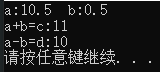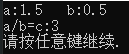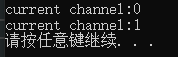# 1.友元函数

• 该函数能访问类声明的非公有部分；
• 该函数位于类的作用域中；
• 该函数必须经由一个对象去激活（有一个this指针）。

#include <iostream>

//自定义一个数值类型，用于测试友元
class Number
{
public:
//通过一个浮点数进行构造
explicit Number(double val) :_value(val) {}
//通过公有接口获取值
double value() const { return _value; }

private:
double _value;

//声明加上friend，即为友元
//定义可以在外部或内部，但是需要在外部做声明以便全局访问该函数
friend Number add(const Number& a, const Number& b)
{
//声明为友元，就可以直接访问类的非公有成员
return Number(a._value + b._value);
}
friend Number sub(const Number& a, const Number& b);
};

//函数的声明，定义可以放cpp中
Number add(const Number& a, const Number& b);
Number sub(const Number& a, const Number& b)
{
return Number(a._value - b._value);
}

int main()
{
Number a(10.5), b(0.5);
Number c = add(a, b); //=11
Number d = sub(a, b); //=10

std::cout << "a:" << a.value() << "\t"
<< "b:" << b.value() << "\n"
<< "a+b=c:" << c.value() << "\n"
<< "a-b=d:" << d.value() << std::endl;

system("pause");
return 0;
}# 2.友元类

#include <iostream>

class Fraction;

//自定义一个数值类型，用于测试友元函数
class Number
{
public:
//通过一个浮点数进行构造
explicit Number(double val = 0.0) :_value(val) {}
//通过公有接口获取值
double value() const { return _value; }

private:
double _value;

//声明加上friend，即为友元
//定义可以在外部或内部，但是需要在外部做声明以便全局访问该函数
friend Number add(const Number& a, const Number& b)
{
//声明为友元，就可以直接访问类的非公有成员
return Number(a._value + b._value);
}
friend Number sub(const Number& a, const Number& b);

friend class Fraction;
};

//函数的声明，定义可以放cpp中
Number add(const Number& a, const Number& b);
Number sub(const Number& a, const Number& b)
{
return Number(a._value - b._value);
}

//自定义一个分数类型，配合Number类测试友元类
class Fraction
{
public:
//通过分子分母构造一个分数
Fraction(const Number& numerator, const Number& denominator)
:_numerator(numerator), _denominator(denominator) {}
//通过公有接口获取分数的浮点值
double value() const
{
//可以通过Number的公有接口访问其值
//return _numerator.value()/_denominator.value();
//也可以把Fraction声明为Number的友元，然后直接访问其值
return _numerator._value / _denominator._value;
}

private:
//分子
Number _numerator;
//分母
Number _denominator;
};

int main()
{
Number a(1.5), b(0.5);
Fraction c(a, b);

std::cout << "a:" << a.value() << "\t"
<< "b:" << b.value() << "\n"
<< "a/b=c:" << c.value() << std::endl;

system("pause");
return 0;
}（在C++Qt框架源码中也大量使用了友元类。）

# 3.友元成员函数

• 声明TV类；
#include <iostream>

class TV;

class Remote
{
public:
};

class TV
{
public:
explicit TV(int channel = 0) :_channel(channel) {}
int channel() const { return _channel; }

private:
int _channel;
};

tv._channel += 1;
}

int main()
{
TV tv(0);
Remote remote;
std::cout << "current channel:" << tv.channel() << std::endl;
std::cout << "current channel:" << tv.channel() << std::endl;

system("pause");
return 0;
}# 4.其他

• C++规定，赋值运算符“=”、下标运算符“[]”、函数调用运算符“（）”、成员运算符“->”必须作为成员函数。
• 流插入“<<”和流提取运算符“>>”、类型转换运算符不能定义为类的成员函数，只能作为友元函数。
• 一般将单目运算符和复合运算符（+=,-=,/=,*=,&=,!=,^=,%=,>>=,<<=）重载为成员函数。
• 一般将双目运算符重载为友元函数。

#include <iostream>

class Number
{
public:
//通过一个浮点数进行构造
explicit Number(double val=0.0) :_value(val) {}
//通过公有接口获取值
double value() const { return _value; }

private:
double _value;
//通过运算符<<获取值
friend std::ostream& operator<<(std::ostream& output, Number& n)
{
output << n._value;
return output;
}
};

# 5.参考

08-292802-22383
02-0238
11-091816
01-3122
11-25548
08-2348
10-23330
03-2432
07-2389
07-2426
01-2035
08-24622
08-30543
01-10281
01-108
09-14730
03-1468
06-282877